Tutorials / p5.js Tutorials / For Loops

# For Loops

tutorial p5.js javascript for-loops

Now you know how to write code using functions, variables, and if statements. So far your code has worked by executing each line one after the other: if you want to draw three circles, you’d have to write three separate calls to the `circle` function.

This tutorial introduces `for` loops, which allow you to repeat work without repeating code.

This sketch draws three vertical lines: the first from position `75,0` to `55,height`; the second from position `150,0` to `150,height`; and the third from position `225,0` to `225,height`.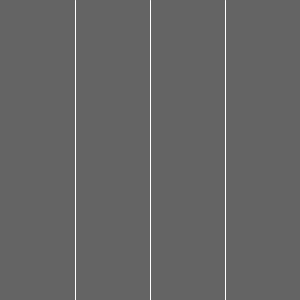As you read this code, try to recognize the pattern in the three lines: the x position of the first line starts at `75`, then increases by `75` for the second line, and stops at `225` for the third line.

When you have a pattern like this (start at a number, increase by a number, stop at a number), you can use `for` loops to follow that pattern to repeat code.

# For Loops

To write a `for` loop, first type the `for` keyword, and then in parentheses `()` provide three things:

• Declare a variable to keep track of your pattern, and initialize it to the number your pattern starts at: `let lineX = 75;`
• Write a test that evaluates to a boolean value of `false` whenever the pattern should stop: `lineX <= 225;`
• Reassign the variable so that it follows the pattern: `lineX = lineX + 75;` (which can be shortened to `lineX += 75`)

Then inside curly brackets `{}`, write the code that uses your variable to follow the pattern. Putting it all together, it looks like this:

This is new syntax, so let’s go over it piece by piece:

• `let lineX = 75;` creates a loop variable with a value of `75`. This only happens once, at the very beginning of the loop.
• `lineX <= 225;` decides when to keep looping. This test is evaluated every step (which is called an iteration) of the pattern, at the beginning of the iteration. Whenever the test evaluates to `false`, the pattern is over and the loop stops iterating.
• `lineX += 75` updates the loop variable. This happens at the end of every iteration, after the body of the loop has run.
• `line(lineX, 0, lineX, height);` uses the `lineX` variable to draw a line each iteration of the loop.

At the end of each iteration (when the code reaches the closing curly bracket `}`), a couple things happen:

• The code executes your reassignment statement to update the loop variable.
• Then the code jumps back to the beginning of the `for` loop.
• The check is evaluated, and if it’s `true`, the body of the loop is executed again. If it’s `false`, the loop exits and skips over the body.

This might seem like a lot to take in, but you can think about it as a few steps:

1. A loop variable is created and initialized to the first number in the pattern.
2. The check is evaluated, and if it’s `false`, the loop exits and its body is skipped. If it’s `true`, then the body is executed.
3. After the body executes, the loop variable is updated.
4. Then the code jumps back to the beginning of the loop and performs the check again.

# The Benefit of For Loops

Three lines might not seem very interesting, but `for` loops make it easier to make more complicated patterns. For example, what if you wanted to draw nine lines instead of three lines?

You could write code that draws each line manually:

This works, but it’s pretty annoying to work with code like this.

Read the code and try to notice the pattern: the first line has an `x` value of `30`, which increases by `30` each step, and ends at `270`. Since you have a pattern, that means you can use a `for` loop to do this in a more manageable way:

This code uses a `for` loop to create a pattern where `lineX` starts at `30`, increases by `30` each iteration, and stops when `lineX` is greater than `270`. During each step of the pattern, the code draws a vertical line using the `lineX` variable.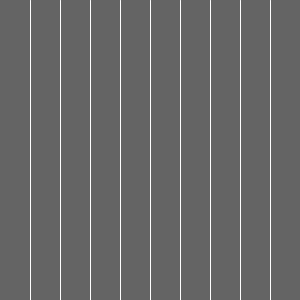Here’s the payoff: what if you wanted to draw 99 lines? You can use a `for` loop to draw the pattern for you instead of writing 99 lines of code: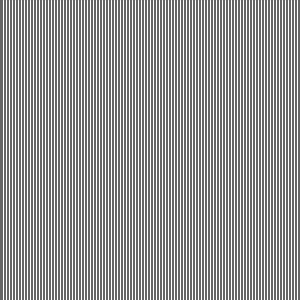To understand the power of `for` loops, imagine writing this sketch without them!

# Nested For Loops

You can put any code inside a `for` loop- including another `for` loop!

For example, let’s start with a program that draws a row of circles:

This sketch uses a `for` loop to draw three circles: one at `75,150`, another at `150,150`, and a third one at `225,150`.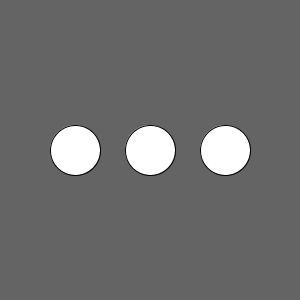You can think of this `for` loop as a single unit that draws a row of circles. What if you wanted to draw three rows of circles?

You could use three separate `for` loops, one for each row: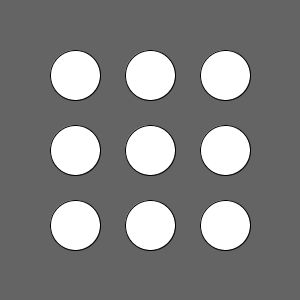This code works, but it’s going to be annoying if you want to add another circle to each row, or change the diameter of the circles: you’d have to change the code in three different places.

Looking at the vertical position of each row, you might notice a pattern: it starts at `75`, increases by `75` each step, and ends at `225`. This sounds like a job for another `for` loop!

The outer loop creates a `circleY` variable and iterates three times. During each iteration of the outer loop, the inner loop creates a `circleX` variable and iterates three times. The end result is nine circles in a grid, or three rows of three circles each.

Try thinking about the inner `for` loop as a single unit, and the outer `for` loop as a loop that executes that unit multiple times. To make that explicit, you could put the inner `for` loop inside a function, which gets called from the outer loop:

This code does the same thing as before, but it encapsulates the inner `for` loop inside the `drawCircleRow` function. The `for` loop inside the `draw` function calls the `drawCircleRow` function three times. The `drawCircleRow` function uses its own `for` loop to draw a row of circles.

# Indexes

The above examples used loop variables that were then used directly in the body of the `for` loop, like `lineX` and `circleX`.

Another common approach you’ll see is to use index loop variables (often named `i` or `j`) that represent how many times the loop should iterate.

Here’s the line example from above, using an index loop variable:This code still does the same thing, but it’s more obvious how many lines will be drawn by reading the `for` loop. The pattern is created by basing the `lineX` variable off the `i` variable, and increasing the `i` variable by `1` each iteration of the loop.

This approach also makes it easier to apply multiple effects to your pattern. For example, this code increases the thickness of the lines as they get closer to the right side of the window: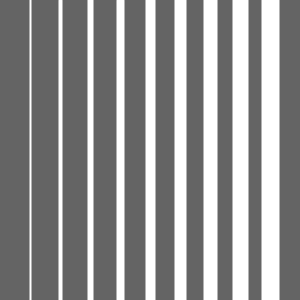Whether you use an index variable or not is up to you and what seems easier to read. You’ll see both approaches in other people’s code.

# Summary

A `for` loop lets you repeat a pattern without writing the same line of code over and over again. You should use a `for` loop when you have code that uses a pattern that starts at a number, increases by a number, and stops at a number.

A `for` loop inside of another `for` loop is called a nested `for` loop. These are useful when your pattern involves more than one number or if you’re working with grids.

You can use an index variable to base your `for` loop on which step of the pattern you’re on, which makes it easier to apply multiple effects at once.

# Cheat Sheet# Homework

• Write a program that gives you the total of 1+2+3+4+…+100. Hint: try it without `for` loops first and try to find a pattern.
• Draw a 10x10 grid that fills up the window, no matter what size the window is.
• Write a program that draws a flower with 8 petals. Hint: try it without `for` loops first and try to find a pattern.